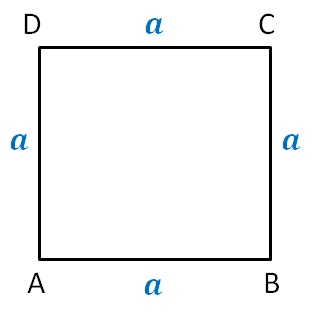Perimeter as variable

Chapter 11 Class 6 Algebra
Concept wise

Let's consider a square ABCDA Square has 4 equal sides

Let side of square = a

So, AB = BC = CD = AD = a

Thus,

Perimeter = Sum of sides

= a + a + a + a

= 4a

So, we can write

Perimeter of square = 4a

where a is the side of square

Learn in your speed, with individual attention - Teachoo Maths 1-on-1 Class